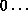## 5.4.1. Communicator AccessorsUp: Communicator Management Next: Communicator Constructors Previous: Communicator Management

The following are all local operations.

MPI_COMM_SIZE(comm, size)
[ IN comm] communicator (handle)
[ OUT size] number of processes in the group of comm (integer)

int MPI_Comm_size(MPI_Comm comm, int *size)

MPI_COMM_SIZE(COMM, SIZE, IERROR)
INTEGER COMM, SIZE, IERROR

[] Rationale.

This function is equivalent to accessing the communicator's group with MPI_COMM_GROUP (see above), computing the size using MPI_GROUP_SIZE, and then freeing the temporary group via MPI_GROUP_FREE. However, this function is so commonly used, that this shortcut was introduced. ( End of rationale.)

This function indicates the number of processes involved in a communicator. For MPI_COMM_WORLD, it indicates the total number of processes available (for this version of MPI, there is no standard way to change the number of processes once initialization has taken place).

This call is often used with the next call to determine the amount of concurrency available for a specific library or program. The following call, MPI_COMM_RANK indicates the rank of the process that calls it in the range fromsize-1, where size is the return value of MPI_COMM_SIZE. ( End of advice to users.)
MPI_COMM_RANK(comm, rank)
[ IN comm] communicator (handle)
[ OUT rank] rank of the calling process in group of comm (integer)

int MPI_Comm_rank(MPI_Comm comm, int *rank)

MPI_COMM_RANK(COMM, RANK, IERROR)
INTEGER COMM, RANK, IERROR

[] Rationale.

This function is equivalent to accessing the communicator's group with MPI_COMM_GROUP (see above), computing the rank using MPI_GROUP_RANK, and then freeing the temporary group via MPI_GROUP_FREE. However, this function is so commonly used, that this shortcut was introduced. ( End of rationale.)

This function gives the rank of the process in the particular communicator's group. It is useful, as noted above, in conjunction with MPI_COMM_SIZE.

Many programs will be written with the master-slave model, where one process (such as the rank-zero process) will play a supervisory role, and the other processes will serve as compute nodes. In this framework, the two preceding calls are useful for determining the roles of the various processes of a communicator. ( End of advice to users.)
MPI_COMM_COMPARE(comm1, comm2, result)
[ IN comm1] first communicator (handle)
[ IN comm2] second communicator (handle)
[ OUT result] result (integer)

int MPI_Comm_compare(MPI_Comm comm1,MPI_Comm comm2, int *result)

MPI_COMM_COMPARE(COMM1, COMM2, RESULT, IERROR)
INTEGER COMM1, COMM2, RESULT, IERROR

MPI_IDENT results if and only if comm1 and comm2 are handles for the same object (identical groups and same contexts). MPI_CONGRUENT results if the underlying groups are identical in constituents and rank order; these communicators differ only by context. MPI_SIMILAR results if the group members of both communicators are the same but the rank order differs. MPI_UNEQUAL results otherwise.Up: Communicator Management Next: Communicator Constructors Previous: Communicator Management